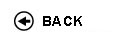Politecnico di Torino
Academic Year 2017/18
04LSILM, 02LSILP, 04LSIOA, 04LSIOD, 04LSIPC
Mathematical methods
1st degree and Bachelor-level of the Bologna process in Computer Engineering - Torino
1st degree and Bachelor-level of the Bologna process in Electronic And Communications Engineering - Torino
1st degree and Bachelor-level of the Bologna process in Computer Engineering - Torino
Espandi...
 Teacher Status SSD Les Ex Lab Tut Years teaching Santacroce MarinaA2 SECS-S/06 80 20 0 0 6
 SSD CFU Activities Area context MAT/05MAT/06 64 A - Di baseA - Di base Matematica, informatica e statisticaMatematica, informatica e statistica
 Subject fundamentals The course aims at completing the students' education in basic mathematics, by introducing the theory of analytic functions, distributions, Fourier and Laplace transforms, and discrete and continuous probability. Such topics play an essential role in engineering applications. Examples and motivation will be drawn from problems in engineering, offering further insights. Expected learning outcomes a) Knowledge and understanding Students are taught some basic mathematical notions and tools for solving various problems ranging from signals analysis to the study of random phenomena. The theory of distributions provides a general language which enables to deal with signals arising in impulsive or discontinuous phenomena: this theory is the natural setting for the study of the Fourier and Laplace transforms. Students learn the techniques for the computation of the transforms of the main distributions: delta Dirac, Dirac comb, and piecewise regular functions included. Complex analysis is the proper setting for the theory of the Laplace transform and is the advanced tool for the analysis of singular phenomena and for the computation of integrals. Moreover, students are provided with the main probabilistic tools necessary for solving problems under uncertainty. They learn how to deal with random phenomena and with the variables involved in them. b) Applying knowledge and understanding At the end of the course students will be able to apply the analytical techniques required for the analysis of the signals of any nature (impulsive, erratic, etc.). Also, they will be expected to have acquired the skills to evaluate the probability of outcomes and extrapolate information useful in solving problems in electronic and telecommunication engineering. The ability to apply the gained knowledge will be verified through class exercises. Prerequisites / Assumed knowledge Students are required to be familiar with the notions and tools of the mathematics courses of the first two years: these include differential and integral calculus of one or several variables. Contents 1. (27h) Function theory of complex variable: differentiability, Cauchy-Riemann equations, line integrals. Cauchy theorem, Cauchy integral formula, Taylor series of analytic functions, Laurent series. Residue theorem, computation of residues and application to the calculation of integrals. 2. (15h) Theory of distributions: definitions and basic operations (algebraic operations, translation, rescaling, derivatives), Dirac delta, p.v.(1/t), Dirac comb. Convolution of functions and distributions. 3. (18h) Fourier and Laplace transforms of functions and tempered distributions: definitions and properties, inverse transforms, inversion formula. Notable transforms. 4. (10h) Combinatorics, probability measures and related elementary properties. Conditional probability and independence. 5. (15h) Discrete and continuous random variables, distribution of a random variable. Notable examples. 6. (15h) Expectation, joint distribution, independence correlation, conditional expected values. Delivery modes Exercises will cover the topics of the lectures. Some of them will be carried out by the teacher at the blackboard, others will actively involve the students. Texts, readings, handouts and other learning resources Lecture notes will be available in the course web page. Recommended textbook in probability: Ross, S. 'A first course in Probability', Pearson Ed., 2014 (or any other edition). Assessment and grading criteria The final exam is written. An oral exam is optional on students' request or at the discretion of the instructor. The written exam is two hour long. Students are allowed to use only a non-programmable calculator and the formulae sheets provided by the instructors. The written exam is composed of two parts: 1. ten multiple-choice quizzes, six of which in analysis and four in probability; 2. two exercises, one in analysis and one in probability, composed of different questions. For each quiz, four possible answers are shown, only one of which is correct. The goal of the multiple choice test is to verify the understanding of the fundamental basic concepts of the analysis and probability parts. Each answer to the test is evaluated 1 point if correct and 0 otherwise. Thus the maximum score to the test is 10. The scope of the exercises of the second part is to verify the knowledge and capability to treat problems involving complex analysis, distributions theory, Fourier and Laplace transforms, probability, random variables and expected values. The exercise in analysis is evaluated maximum 13 points, the one in probability 9 points. To pass the written part of the exam students have to totalize at least 18/30, with at least 4/30 in probability and at least 6/30 in analysis. If the sum of the two parts of the exam is less or equal to 30, it represents the final mark. If it is 31 or 32, the final mark is 30 or 30 with honor (30L) respectively. Only students who passed the written exam can ask to be admitted to the oral exam. In particular, if an oral exam is asked and performed, it becomes part of the evaluation together with the written part. Depending on the performance of the student, the final mark could be less, equal or greater than the total score of the written exam. Programma definitivo per l'A.A.2017/18© Politecnico di Torino
Corso Duca degli Abruzzi, 24 - 10129 Torino, ITALY